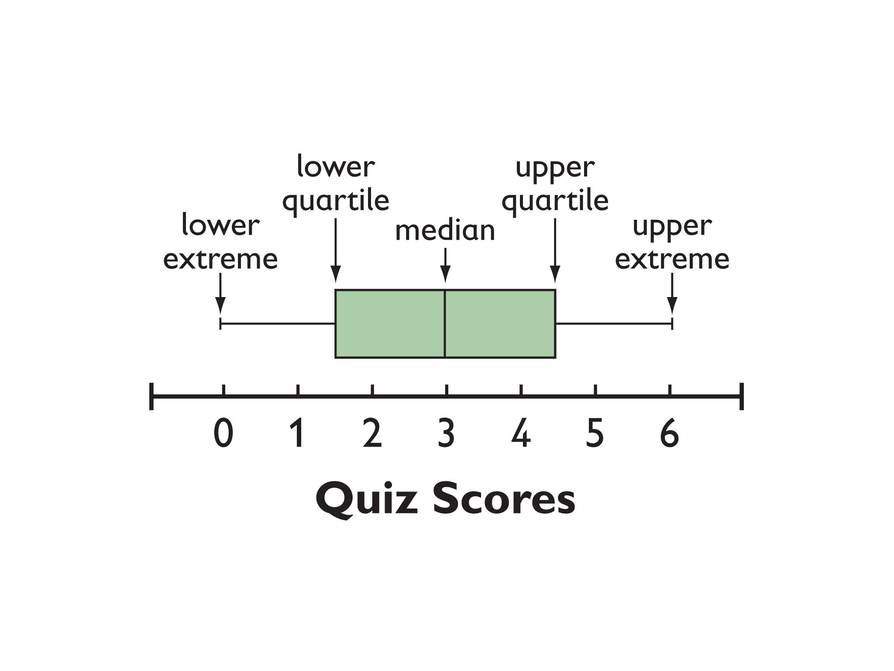# Calculating The Five-Number Summary & Interquartile Range (IQR)

Student View (Opens in new window)## Instructor Overview

Students make a box plot for their typical-sixth-grader data from Lesson 7 and write a summary of what the plot shows.

Using the line plot from Lesson 4, students construct a box plot. Students learn how to calculate the five-number summary and interquartile range (IQR). Students apply this knowledge to the data used in Lesson 7 and describe the data in terms of the box plot. Class discussion focuses on comparing the two graphs and what they show for the sets of data.

# Key Concepts

A box-and-whisker plot, or box plot, shows the spread of a set of data. It shows five key measures, called the five-number summary.

• Lower extreme: The smallest value in the data set
• Lower quartile: The middle of the lower half of the data, and the value that 25% of the data fall below
• Median: The middle of the data set
• Upper quartile: The middle of the upper half of the data, and the value that 25% of the data are above
• Upper extreme: The greatest value in the data set

This diagram shows how these values relate to the parts of a box plot.The length of the box represents the interquartile range (IQR), which is the difference between the lower and upper quartile.

A box plot divides the data into four equal parts. One quarter of the data is represented by the left whisker, two quarters by each half of the box, and one quarter by the right whisker. If one of these parts is long, the data in that quarter are spread out. If one of these quarters is short, the data in that quarter are clustered together.

# Goals and Learning Objectives

• Learn how to construct box plots, another tool to describe data.
• Learn about the five-number summary, interquartile range, and how they are related to box plots.
• Compare a line plot and box plot for the same set of data.